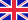•# Quotient Space

Autor: Lambert M. Surhone

High Quality Content by WIKIPEDIA articles! In topology and related areas of mathematics, a quotient space (also called an identification space) is, intuitively speaking, the result of identifying or 'gluing together' certain points of a given space. The... Viac o knihe

Na objednávku, dodanie 2-4 týždne

25.42 €

bežná cena: 29.90 €

## O knihe

High Quality Content by WIKIPEDIA articles! In topology and related areas of mathematics, a quotient space (also called an identification space) is, intuitively speaking, the result of identifying or 'gluing together' certain points of a given space. The points to be identified are specified by an equivalence relation. This is commonly done in order to construct new spaces from given ones. Suppose X is a topological space and ~ is an equivalence relation on X. We define a topology on the quotient set X/~ (the set consisting of all equivalence classes of ~) as follows: a set of equivalence classes in X/~ is open if and only if their union is open in X. This is the quotient topology on the quotient set X/~. Equivalently, the quotient topology can be characterized in the following manner: Let q : X X/~ be the projection map which sends each element of X to its equivalence class. Then the quotient topology on X/~ is the finest topology for which q is continuous.

• Vydavateľstvo: Betascript Publishers
• Rok vydania: 2009
• Formát: Paperback
• Rozmer: 220 x 150 mm
• Jazyk: Anglický jazyk
• ISBN: 9786130304140

Generuje redakčný systém BUXUS CMS spoločnosti ui42.